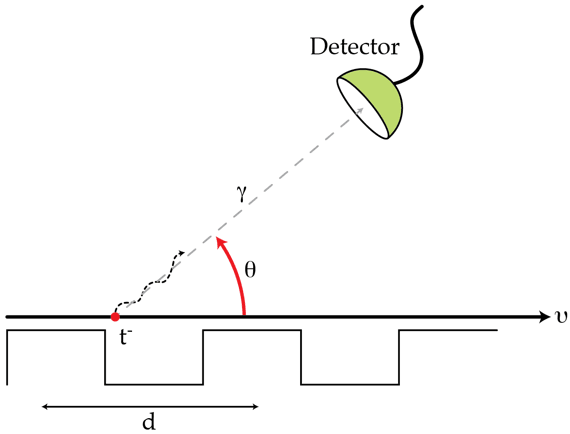# Colorful Electrons

A narrow beam of electrons passes close to the surface of a large metallic mirror with a diffraction grating of period $d=2~\mu\text{m}$ inscribed on it as shown in the figure. The electrons move with $v=0.9c$ ($c$ is the speed of light) at right angles to the lines of the grating. As a result of the etchings, the electrons may emit visible radiation whose wavelength depends on the angle of observation $\theta$. At which angle $\theta$ in degrees will the observed radiation be green? Assume that the velocity of the electrons is not affected by the mirror.Details and assumptions

Assume that green light has wavelength $\lambda_{g}=0.55~\mu \text{m}.$)

×